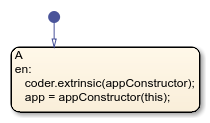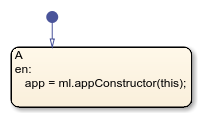this

Access chart data during simulation

Description

example

this provides external MATLAB® code, such as functions and apps, access to chart data during simulation.

• For charts in Simulink® models, external MATLAB code can access inputs, outputs, and local data.

• For standalone charts in MATLAB, external MATLAB code can access local data and call step, input event functions, and graphical and MATLAB functions in the chart. For more information, see Execute a Standalone Chart.

Note

In charts in Simulink models, the keyword this is supported only as an argument to external MATLAB code. Any other use of the keyword in the chart results in a compile-time error.

Examples

expand all

Create a bidirectional connection between a Stateflow® chart and a MATLAB app created in App Designer. Call the app as an extrinsic function using this as an argument to the constructor. In the app, create a custom property to interface with the chart during simulation. In the chart, store the value returned by the function call to the app as a local data object.

In a chart that uses MATLAB as the action language, enter:

coder.extrinsic(appConstructor);
app = appConstructor(this);In a chart that uses C as the action language, enter:

app = ml.appConstructor(this);For additional examples that illustrate this workflow, see Model a Power Window Controller and Simulate a Media Player.

Modify the value of the local data x while debugging a standalone Stateflow chart in MATLAB.

At the debugging prompt, enter:

this.x = 7

Note

When debugging a chart in a Simulink model, you can access all Stateflow data directly at the debugging prompt. For more information, see View and Modify Data in the MATLAB Command Window.

Tips

• Do not use the keyword this to access chart data after simulation has stopped.

• Calling an external function named this from a chart disables the keyword this throughout the chart. To use the keyword, rename the extrinsic function.

Introduced in R2020b

Stateflow DocumentationGet trial now